# Simulation and design of Three phase Rectifier in Simulink MATLAB

In this tutorial, I will explain the working and implementation of a three phase rectifier. As we have implemented a single phase rectifier in previous tutorials it will be really easy for you to understand the working of a three phase rectifier. At the start a brief and concise introduction of rectifiers and three phase rectifiers is provided along with its types. After that proper implementation of three phase rectifiers on MATLAB’s simulink is explained along with the working of each step and purpose of each block used and parameters settings of each block.

## Introduction to rectifiers and three phase rectifiers

In electrical/electronics engineering, the word rectifier is recognized as a device which can convert an input AC voltage to DC voltage. In every house the power supply provided by the government is three phase Alternating (AC) but most of our devices like laptops and mobile phones uses Direct voltage (DC voltage) for their charging. Hence a three phase rectifier is now a days used in almost every house and is a very common device to be used. Now lets talk about different types of rectifiers.

## Types of rectifier

Depending on the output DC voltage:

• Half wave rectifier
• Full wave rectifier

Depending on the input phase voltage

• Single phase rectifier
• Three phase rectifier

We have already discussed the former two types of rectifiers in previous tutorial, now we will discuss the later two types.

### Single phase rectifier

Rectifiers whose input voltages are single phase AC voltage are known as single phase rectifiers. The output of such a circuit will be high pulsating DC voltage. A waveform of single phase AC voltage is shown in figure below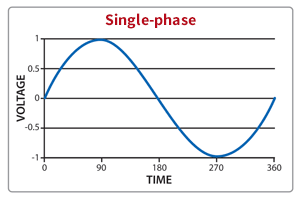Figure 1: Single phase AC

### Three phase rectifier

Those rectifiers the input of which is a three phase AC voltage are known as three phase rectifiers. The output of three phase rectifiers is a low pulsating DC. A three phase AC voltage is shown in the figure below,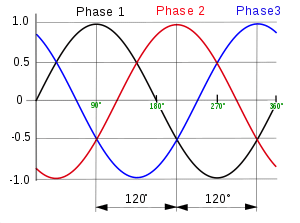Figure 2: Three phase AC

## Simulation of Three phase Rectifier in Simulink MATLAB

Lets’ now commence our discussion of implementing the three phase inverter on MATLAB’s simulink. Open MATLAB and then open simulink and create a blank model as we have been doing previously and save it for future use. Open the library browser and go to the simscape section in library browser as shown in the figure below,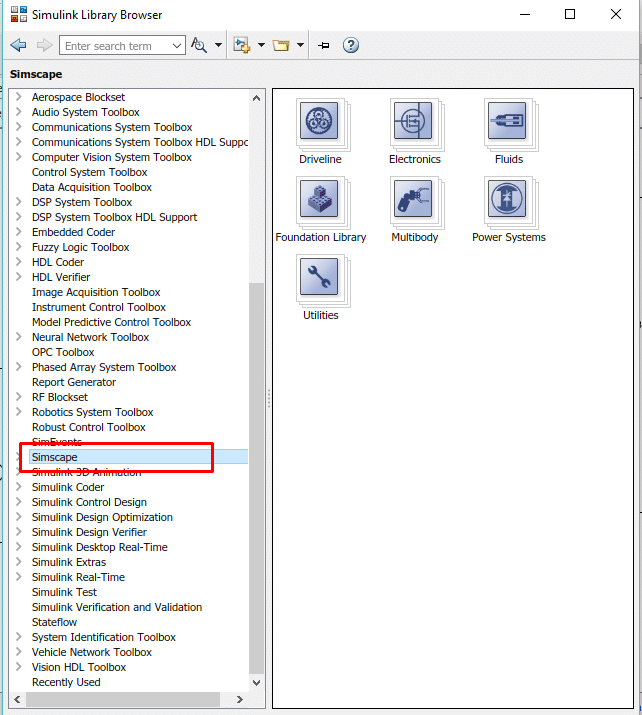Figure 3: Simscape

In this block select the power system block as shown in the figure below,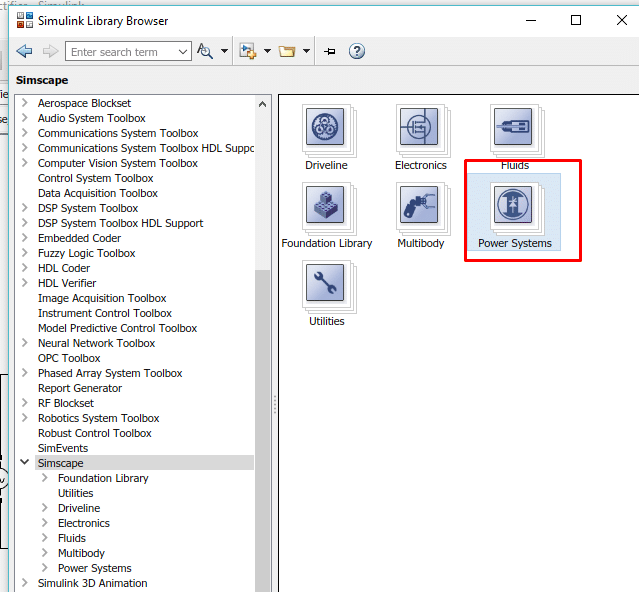Figure 4: Power system

In power system block select the special technology block as shown in the figure below,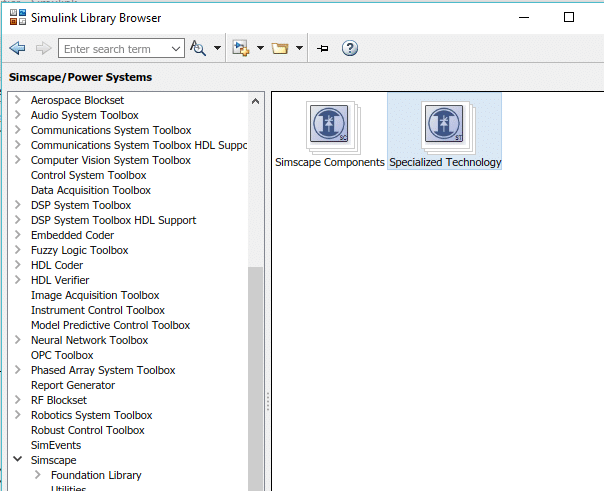Figure 5: Special Technology

After that select the fundamental blocks where almost all the blocks we will be needing in this tutorial resides. Refer to the figure below to see fundamental blocks.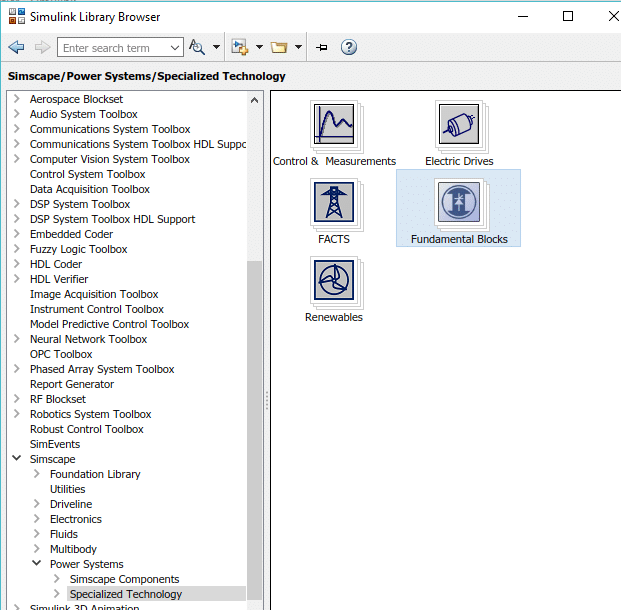Figure 6: Fundamental blocks

Inside that section there will be block named power gui, select this block and add it to the model we have created before as shown in the figure below,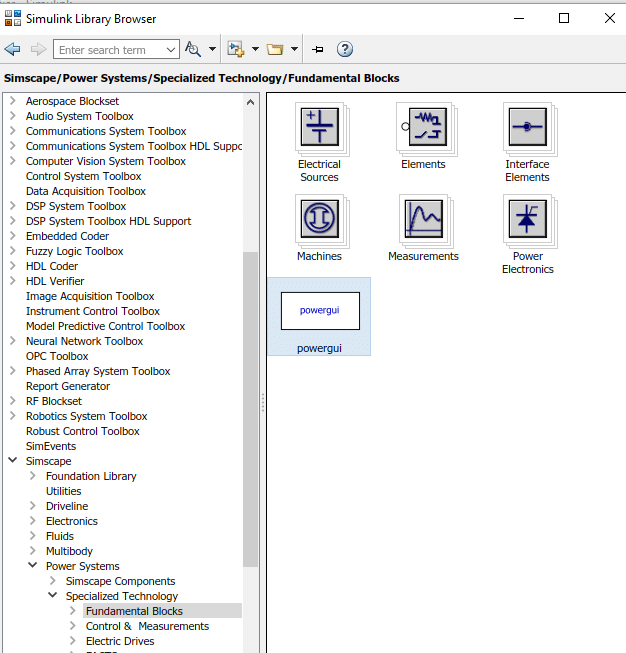Figure 7: Power gui

The function and use of this block is discussed in many of the previous tutorials. After that in fundamental block section select the block named Electrical sources as shown in the figure below,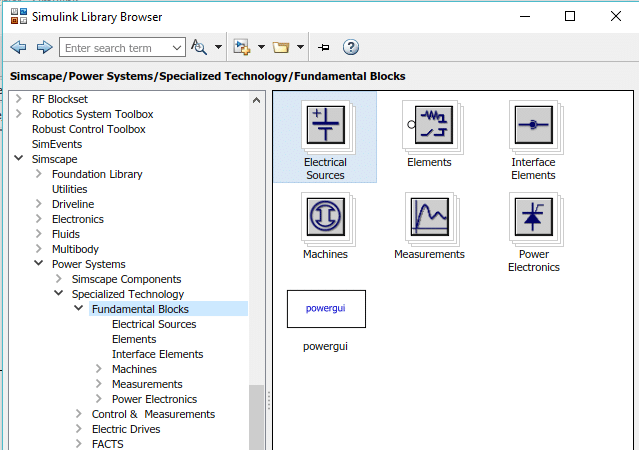Figure 8: Electrical sources

This block contains all the sources needed in power systems. Inside this block select the AC voltage source and place three such sources on the model as shown in the figure below,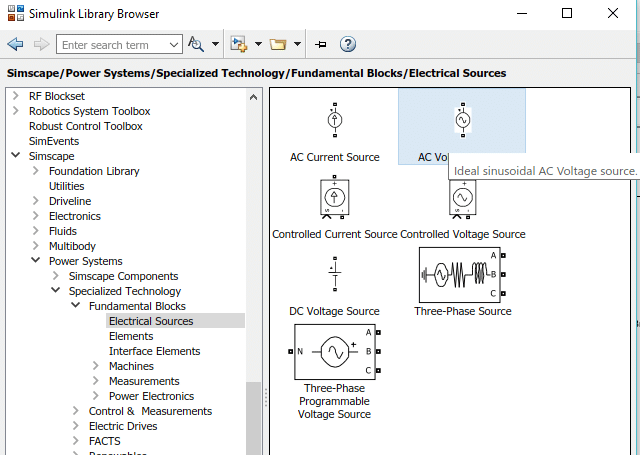Figure 9: AC source

We need three sources because we want to work with three phase AC sources. Now go back to the fundamental block section and select the power electronic section as shown in the figure below,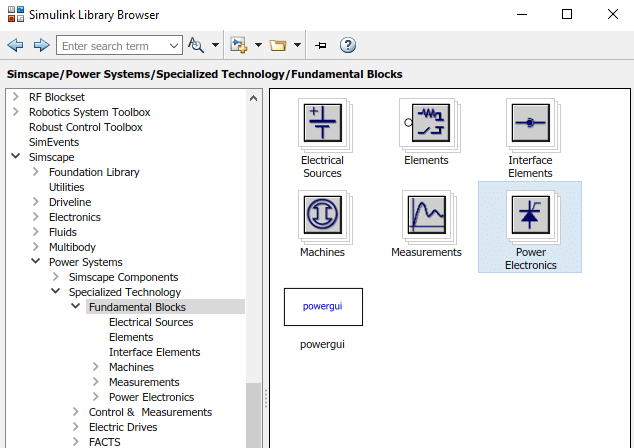Figure 10: Power electronics

In this section select the thyristor block and add six such blocks to the model as shown in the figure below,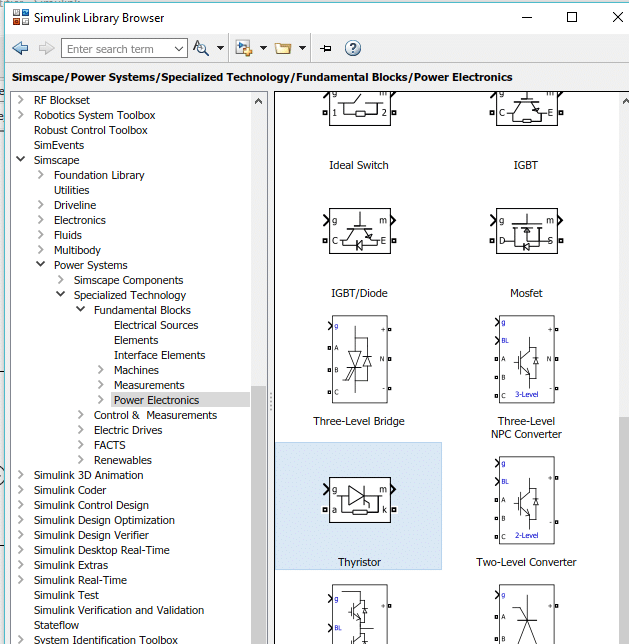Figure 11: Thyristor

We need thyristors in place of diodes when we are working with 3 phase ac source. Now in the library browser go to the simulink section and then select the sources block, as shown in the figure below,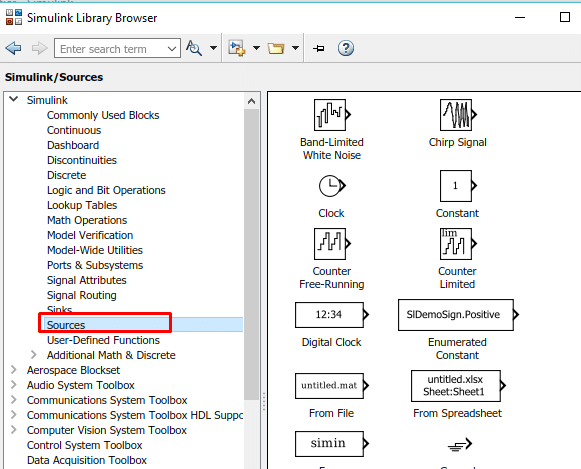From this section select the block named pulse generator and add it to the model as shown in the figure below,Figure 13: Pulse generator

This pulse generator will be used with a pair of the thyristor for turning it on and off. Hence we will need three pulse generator (each for one pair of thyristors). Again go to the simscape’s fundamental blocks section and select the section named as Elements as shown in the figure below,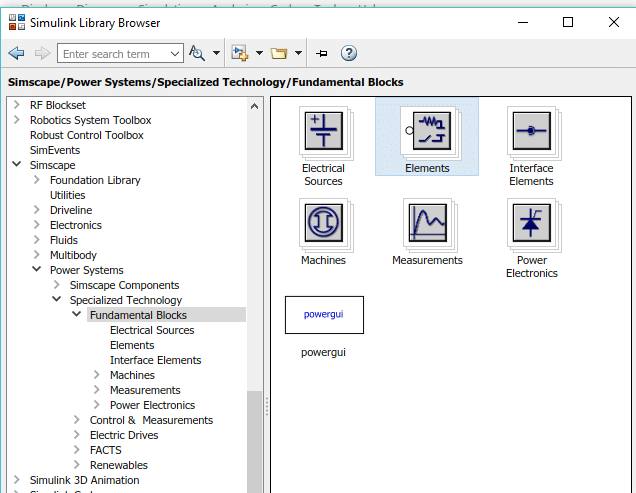Figure 14: Elements

From this section we will need a load for the testing of our 3 phase rectifier. From this section select the series RLC branch and add it to the model as shown in the figure below,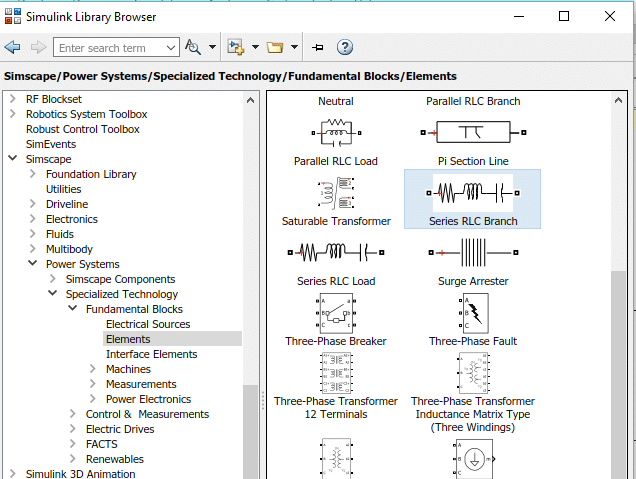Also for the measurement of voltage, go back to fundamental blocks section and select the measurement block where resides all the blocks need for measurement in power electronics or power systems as shown in the figure below,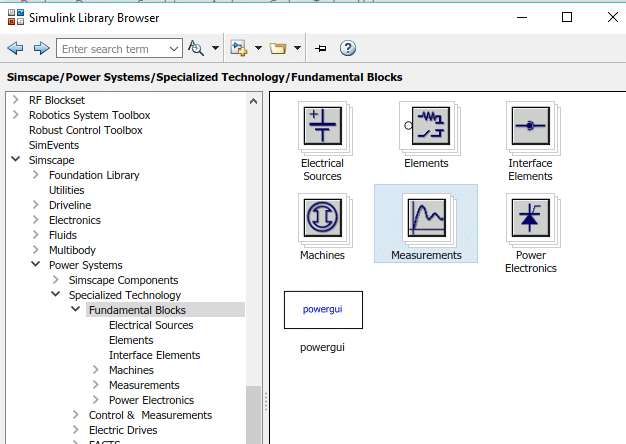Figure 16: Measurement block

In this section we will we need a block for the measurement of voltage hence select the block named as voltage measurement as shown in the figure below,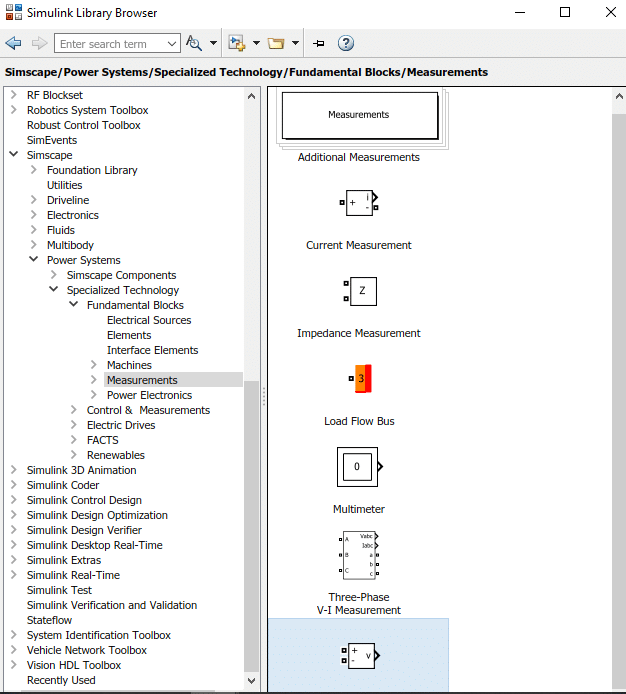Figure 17: Voltage measurement block

Also we need some oscilloscope to view the output to be a pure dc or not. Hence go the section simulink of library browser and select the section named section. From this section select the block named scope and add it to the model as shown in the figure below,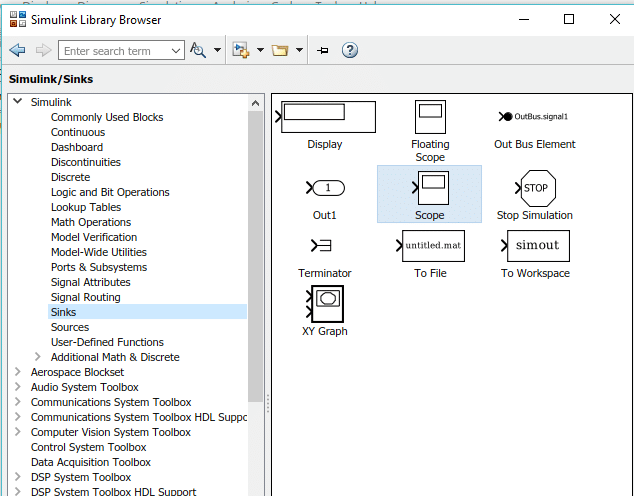Figure 18: Scope

Connect the 6 thyristors in the order we placed in case of 3 phase inverter and connect 3 AC voltage source to the three junctions between each pair of thyristor. Set the voltage of source to 230V and phase angle of first block to 0, second block to 120 and third to 240(-120). The block parameter of last source is shown in the figure below,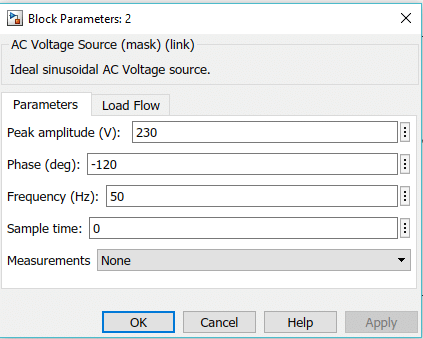Figure 19: AC Voltage source block parameters

Now we need three pulse generator to connect to each pair of thyristors. The connected thyristors are shown in the figure below,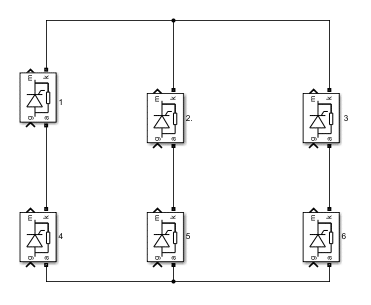Figure 20: Thyristor placed

Connect 1st pulse generator to thyrsitors pair ( 1 , 5 ) 2nd to the thyrystor pair ( 2 , 6 ) and 3rd to the thyrsitor pair ( 3 , 4 ) as shown in the complete block diagram. But first look at the pulse generator block parameters. Set the phase of 1st pulse generator to 30, 2nd to 150 and 3rd to 270 as shown in the figure below,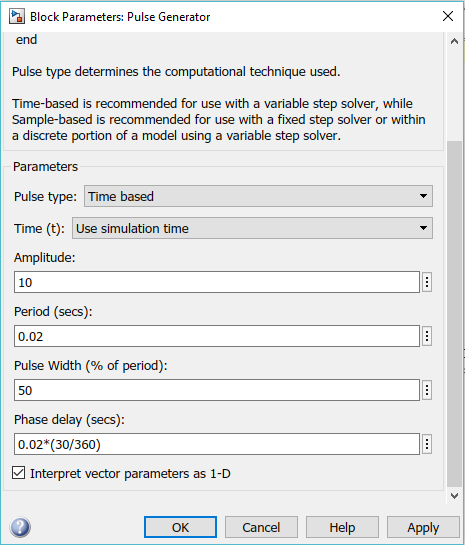Figure 21: Parameters of 1st pulse generator

The complete block diagram of 3 phase rectifier is shown in the figure below,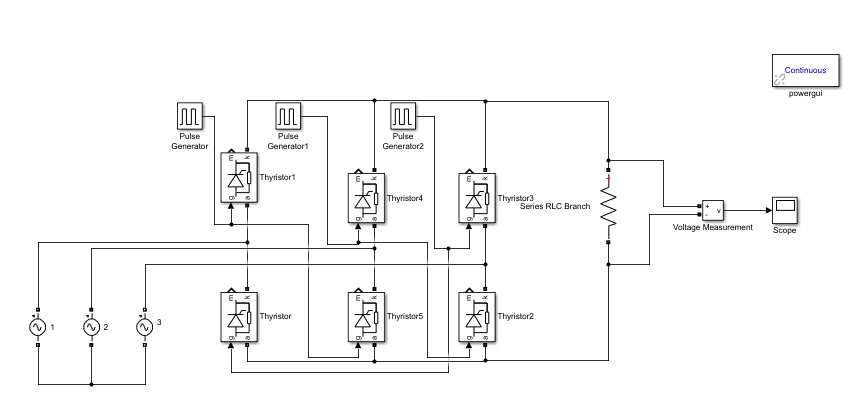Figure 22: Block diagram

Run the model and double click on the scope to see the output of the 3 phase rectifier which should be pulsating dc as shown in the figure below,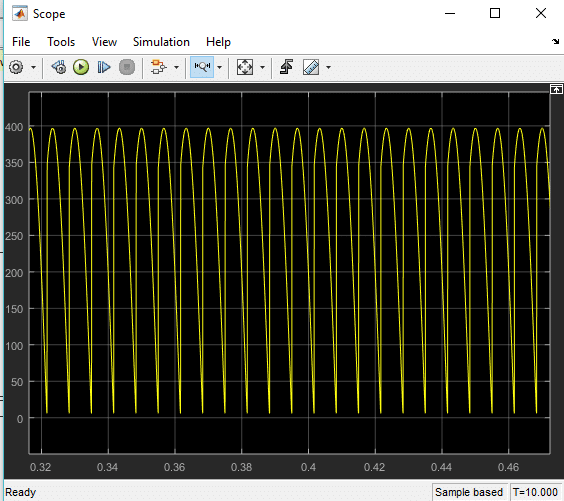Figure 23: Output

The output of the 3 phase rectifier  ( as expected ) is a pulsating DC. The ripples however are way more frequent in 3 phase rectifier as compared to single phase rectifier. We can remove the ripples in rectifier by using RC filters and the output then will look like the one shown in the figure below,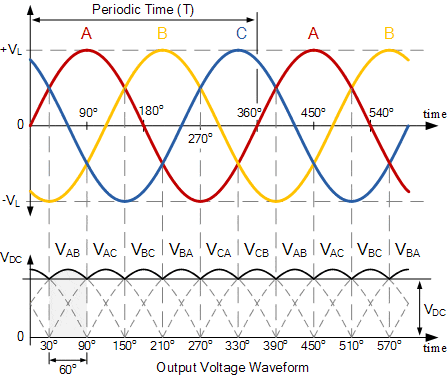Figure 24: Filtered output

### 3 thoughts on “Simulation and design of Three phase Rectifier in Simulink MATLAB”

1. Can i get capaciotr.filter circuit with simulinkmodel